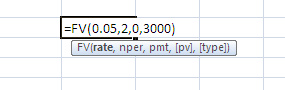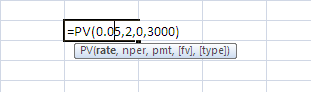## Saturday, September 14, 2013

### Future Value and Present Value Methods

Suppose now you have \$3000 in hand today,it must be worth more than someone promise to give  you \$3000 in the next 2 year.because with  \$3000 in hand today, it can be invested in a bank or fixed income securities(Bond) for example 5% interest rate,so in the next 2 year you will have the principle + interest income.

Formula

FV = PV(1 + r)t

• FV = future value
• PV = present value
• r = period interest rate, expressed as a decimal
• T = number of periods
• Future value interest factor(FVIF) = (1 + r)t

Example:

Method 1

Future Value (FV) = \$3,000(1 + .05)^2 = \$3,306

Method 2

PV = present value =\$3000
r= 5%
T=2 year
Future value interest factor (FVIF) = (1 + r)t can be found in the table below  is the interest rate   5% for 2 years is (1+r)t = 1.102

Future Value (FV) = PV(FVIFi,n) or \$3,000(1.102) = \$3,306

Using Financial calculator

Method 4Present Value: is the The current worth of a future sum of money or stream of cash flows discounted by the Interest rate (Discount rate,Cost of capital, Opportunity cost of capital,Required return)

As we can see in the previous example that \$3000 to be received in the next 2 year probably worth less than \$3000 in hand today.because \$3000 today can be saved with 5% interest rate and  in the 2 years time we will receive \$3,306. so to find the 3000\$ to be received in the next 2 year and how much it's worth today,it have to be discounted with 5% as an opportunity cost.

Formula

PV = FV/(1 + r)t or FV*( 1/(1+r)t)

• FV = future value
• PV = present value
• r = period interest rate, expressed as a decimal
• T = number of periods
• Present value interest factor(PVIF) = 1/(1 + r)t  or Discount Factor

Example:

Method 1

Present Value (PV) = \$3,000/(1 + .05)^2 = \$2,721

Method 2

FV = Future value =\$3000
r= 5%
T=2 year
Present value interest factor(PVIF) or Discount Factor = (1 + r)t can be found in the table below  is the interest rate   5% for 2 years is 1/(1+r)t =0.907

Present Value (PV)FV(PVIFi,n) or \$3,000(0.907) = \$2,721

Method 4